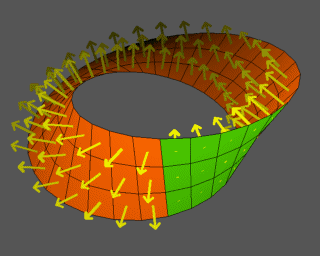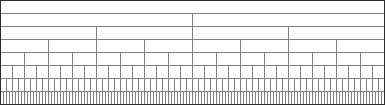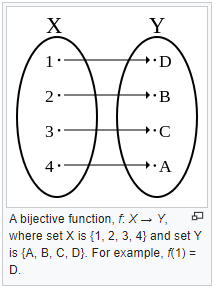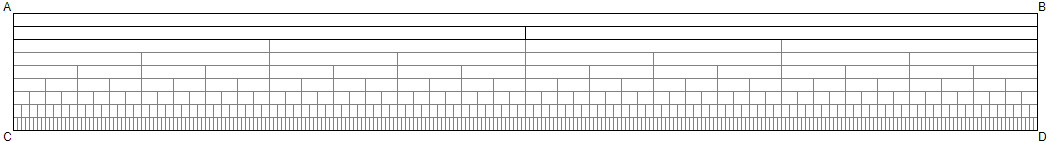Closed Loop Interval OntologyCLOSED LOOP INTERVAL ONTOLOGY        The Digital Integration of Conceptual FormTzimTzum/Kaballah | Loop definition | Home | ORIGINPlease sign in or register Email * Password * Searches selected display Universal conceptual form Aligning the vision Evolving and coalescing Why we do this Ethics / governance / science Homeostatic governance Idealized democracy Reconciliation and integration Holistic view on alternatives Definitions and alternatives Compare alternatives How do we know? How meaning is created Foundational recursive definition Spectrum of levels The closed loop ensemble contains all primary definitions Dimensions of set theory What is a number? Topology of sets How are they constructed? Primary terms Closed Loop framework Hierarchical models Euclid in digital space Foundational method Compositional semantics How it works The integrated science of mind What does it mean? Core terms Constructive elements and building blocks Preserving data under transformation In the beginning Domain and universal A design proposal Mapping the grid From other sources Foundational computation Perfecting political balance Simultaneity or sequence Concrete symbolic representation Science, philosophy and math are defined in concepts Emerging from a pointEquality
What does it mean?

 What does it mean to say a=b when they are obviously not the same thing? They are "the same thing in some regards" - but how do we define or locate those "regards"?

 Equality and the axiomatic method "It depends on what the meaning of is is." - Bill Clinton If we say "a = b and b = a" - what does that mean? Can we build a reliable comprehensive system based on those concepts - when it might not be clear 1) what "a" and "b" actually are, and 2) what it means to say they are equal. We know it works - but we don't really know why. Asking this question is just another open thread for exploration as the moment emerges, and the energy to approach this topic carefully has begun to coalesce. Mon, May 10, 2021 Reference In mathematics and logic, an axiomatic system is any set of axioms from which some or all axioms can be used in conjunction to logically derive theorems. A theory is a consistent, relatively-self-contained body of knowledge which usually contains an axiomatic system and all its derived theorems. An axiomatic system that is completely described is a special kind of formal system. A formal theory is an axiomatic system (usually formulated within model theory) that describes a set of sentences that is closed under logical implication. A formal proof is a complete rendition of a mathematical proof within a formal system.

 What is an axiom? The Wikipedia reference makes a couple of important distinctions Logical axioms Thu, Apr 8, 2021 Reference An axiom, postulate or assumption is a statement that is taken to be true, to serve as a premise or starting point for further reasoning and arguments. The word comes from the Greek axíma, 'that which is thought worthy or fit' or 'that which commends itself as evident.' The term has subtle differences in definition when used in the context of different fields of study. As defined in classic philosophy, an axiom is a statement that is so evident or well-established, that it is accepted without controversy or question. As used in modern logic, an axiom is a premise or starting point for reasoning. As used in mathematics, the term axiom is used in two related but distinguishable senses: "logical axioms" and "non-logical axioms". Logical axioms are usually statements that are taken to be true within the system of logic they define and are often shown in symbolic form (e.g., (A and B) implies A), while non-logical axioms (e.g., a + b = b + a) are actually substantive assertions about the elements of the domain of a specific mathematical theory (such as arithmetic). When used in the latter sense, "axiom", "postulate", and "assumption" may be used interchangeably. In most cases, a non-logical axiom is simply a formal logical expression used in deduction to build a mathematical theory, and might or might not be self-evident in nature (e.g., parallel postulate in Euclidean geometry). To axiomatize a system of knowledge is to show that its claims can be derived from a small, well-understood set of sentences (the axioms), and there may be multiple ways to axiomatize a given mathematical domain. Any axiom is a statement that serves as a starting point from which other statements are logically derived. Whether it is meaningful (and, if so, what it means) for an axiom to be "true" is a subject of debate in the philosophy of mathematics.

 Primitive The Closed Loop is a "constructivist" project. We are "building stuff" - from pieces that we put together to form composite systems and "wholes". One thing we are building is "concepts". What are concepts made out of? A "primitive" or a "primitive notion" is supposedly a lowest-level object or element from which abstract ideas can be/are/should be constructed. Mon, May 10, 2021 Reference In mathematics, logic, philosophy, and formal systems, a primitive notion is a concept that is not defined in terms of previously-defined concepts. It is often motivated informally, usually by an appeal to intuition and everyday experience. In an axiomatic theory, relations between primitive notions are restricted by axioms. Some authors refer to the latter as "defining" primitive notions by one or more axioms, but this can be misleading. Formal theories cannot dispense with primitive notions, under pain of infinite regress (per the regress problem). For example, in contemporary geometry, point, line, and contains are some primitive notions. Instead of attempting to define them, their interplay is ruled (in Hilbert's axiom system) by axioms like "For every two points there exists a line that contains them both".

 Equality (mathematics) Sat, Apr 10, 2021 Reference In mathematics, equality is a relationship between two quantities or, more generally two mathematical expressions, asserting that the quantities have the same value, or that the expressions represent the same mathematical object. The equality between A and B is written A = B, and pronounced A equals B. The symbol "=" is called an "equals sign". Two objects that are not equal are said to be distinct. For example: {\displaystyle x=y}x=y means that x and y denote the same object. The identity {\displaystyle (x+1)^{2}=x^{2}+2x+1}{\displaystyle (x+1)^{2}=x^{2}+2x+1} means that if x is any number, then the two expressions have the same value. This may also be interpreted as saying that the two sides of the equals sign represent the same function. {\displaystyle \{x\mid P(x)\}=\{x\mid Q(x)\}}{\displaystyle \{x\mid P(x)\}=\{x\mid Q(x)\}} if and only if {\displaystyle P(x)\Leftrightarrow Q(x).}{\displaystyle P(x)\Leftrightarrow Q(x).} This assertion, which uses set-builder notation, means that if the elements satisfying the property {\displaystyle P(x)}P(x) are the same as the elements satisfying {\displaystyle Q(x),}{\displaystyle Q(x),} then the two uses of the set-builder notation define the same set. This property is often expressed as "two sets that have the same elements are equal." It is one of the usual axioms of set theory, called axiom of extensionality. ********* Basic properties Substitution property: For any quantities a and b and any expression F(x), if a = b, then F(a) = F(b) (provided that both sides are well-formed). Some specific examples of this are: For any real numbers a, b, and c, if a = b, then a + c = b + c (here, F(x) is x + c); For any real numbers a, b, and c, if a = b, then a ? c = b ? c (here, F(x) is x ? c); For any real numbers a, b, and c, if a = b, then ac = bc (here, F(x) is xc); For any real numbers a, b, and c, if a = b and c is not zero, then a/c = b/c (here, F(x) is x/c). Reflexive property: For any quantity a, a = a. Symmetric property: For any quantities a and b, if a = b, then b = a. Transitive property: For any quantities a, b, and c, if a = b and b = c, then a = c. These three properties make equality an equivalence relation. They were originally included among the Peano axioms for natural numbers. Although the symmetric and transitive properties are often seen as fundamental, they can be deduced from substitution and reflexive properties. ********** Relation with equivalence and isomorphism Main articles: Equivalence relation and Isomorphism Viewed as a relation, equality is the archetype of the more general concept of an equivalence relation on a set: those binary relations that are reflexive, symmetric and transitive. The identity relation is an equivalence relation. Conversely, let R be an equivalence relation, and let us denote by xR the equivalence class of x, consisting of all elements z such that x R z. Then the relation x R y is equivalent with the equality xR = yR. It follows that equality is the finest equivalence relation on any set S in the sense that it is the relation that has the smallest equivalence classes (every class is reduced to a single element). *** In some contexts, equality is sharply distinguished from equivalence or isomorphism. For example, one may distinguish fractions from rational numbers, the latter being equivalence classes of fractions: the fractions "1/2 "and "2/4" are distinct as fractions (as different strings of symbols) but they "represent" the same rational number (the same point on a number line). This distinction gives rise to the notion of a quotient set. *** Similarly, the sets {\displaystyle \{{\text{A}},{\text{B}},{\text{C}}\}}{\displaystyle \{{\text{A}},{\text{B}},{\text{C}}\}} and {\displaystyle \{1,2,3\}}{\displaystyle \{1,2,3\}} are not equal sets — the first consists of letters, while the second consists of numbers — but they are both sets of three elements and thus isomorphic, meaning that there is a bijection between them. For example {\displaystyle {\text{A}}\mapsto 1,{\text{B}}\mapsto 2,{\text{C}}\mapsto 3.}\text{A} \mapsto 1, \text{B} \mapsto 2, \text{C} \mapsto 3. However, there are other choices of isomorphism, such as {\displaystyle {\text{A}}\mapsto 3,{\text{B}}\mapsto 2,{\text{C}}\mapsto 1,}\text{A} \mapsto 3, \text{B} \mapsto 2, \text{C} \mapsto 1, and these sets cannot be identified without making such a choice — any statement that identifies them "depends on choice of identification". This distinction, between equality and isomorphism, is of fundamental importance in category theory and is one motivation for the development of category theory. https://en.wikipedia.org/wiki/Equivalence_relation

 Bijection One to one correspondenceSat, Apr 10, 2021 Reference In mathematics, a bijection, bijective function, one-to-one correspondence, or invertible function, is a function between the elements of two sets, where each element of one set is paired with exactly one element of the other set, and each element of the other set is paired with exactly one element of the first set. There are no unpaired elements. In mathematical terms, a bijective function f: X ? Y is a one-to-one (injective) and onto (surjective) mapping of a set X to a set Y. The term one-to-one correspondence must not be confused with one-to-one function (an injective function; see figures).